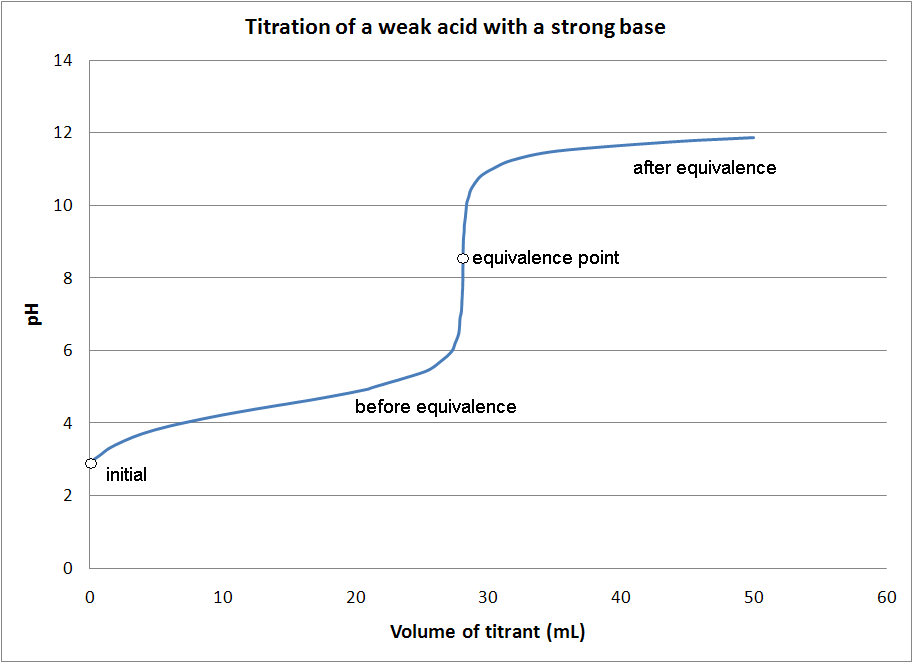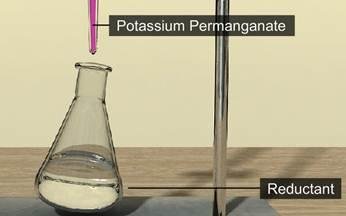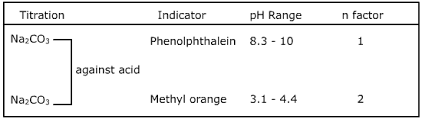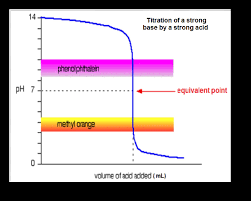# Volumetric Analysis

## Volumetric Analysis Titration in Chemistry

The analytical method wherein the concentration of a substance in a solution is estimated by adding exactly the same number of equivalents of another substance present in a solution of known concentration is called volumetric analysis.
This is the basic principle of titration. Another name for volumetric analysis is titrimetric analysis.

The substance whose solution is used to estimate the concentration of the unknown solution is called titrant. Titrate is the substance whose concentration is to be estimated.

The volumetric analysis can be classified into three types:

• 1. Simple titration

• 2. Back titration

• 3. Double titrations

• SIMPLE TITRATION

The main aim of simple titration is to find the concentration of an unknown solution with the aid of the known concentration of another solution. Simple titration can again be classified into four different types:

• • Acid-base titrations

• • Redox titrations

• • Precipitation titrations and

• • Complexometric titrations

• Acid-base titrations

This type of titration is used to find the concentration of an acid in a solution (or vice-versa). The estimated value of acid in a solution can be determined by adding a solution of standard base (or vice-versa). A suitable indicator is added drop-by-drop to the solution whose concentration is to be estimated. This helps in detecting the equivalence point.

An acid-base indicator gives different colors of different compounds. An indicator is chosen in a particular titration based on the pH-range of the indicator and the pH change near the equivalence point.Redox Titrations

An oxidant can be estimated by adding reductant in redox titrations (or vice-versa). An example of this is; Fe2+ ions can be estimated by titration against acidified KMnO4 solution when Fe2+ ions are oxidized to Fe3+ ions and KMnO4 is reduced to Mn2+ in an acidic medium. Also, KMnO4 is a self-indicator. At the equivalence point, it can be noticed that it discharges a purple color.

Mn + 8H+ + 5e → Mn2+ + 4H2O
Fe2+ → Fe3+ + e] × 5
Mn + 8H+ + 5Fe2+ → Mn2+ + 5Fe3+ + 4H2O
(n=5) (n=1)Acidified KCr2O7 can also be employed instead of KmnO4. Other redox titrations are iodimetry, iodometry etc.

Iodimetry

Here, the process of titration involves free iodine. There is a direct estimation of iodine. Such a titration is called iodimetry. The process basically involves the titration of iodine solution with a known amount of sodium thiosulphate solution.

I2 + 2Na2S2O3 → 2NaI + Na2S4O6

Iodometry

This is known to be an indirect method of estimation of iodine. Here, an oxidizing agent is made to react with an excess of solid KI. The oxidizing agent oxidizes I to I2. The next step is to make the liberated I2 to react with Na2S2O3 solution.

Precipitation Titrations

In a titration of this form, a compound of very low solubility is formed by combining cations and anions. A solid residue can be separated out.

For example,

AgNO3 + NaCl → AgCI¯ (white) + NaNO3
BaCI2 + H2SO4 → BaSO4¯ (white) + 2HCI

Complexometric Titrations

The titrate combines with the titrant which results in the formation of complex salts in this type of titration. The complex salts may or may not be soluble. For example,

CuSO4 + 4NH4OH → [Cu (NH3)4] SO4 + 4H2O
AgNO3 + 2KCN → K [Ag (CN) 2] + KNO3

Back Titration

Let us make an assumption that we have an impure solid substance ‘C'. It is required to calculate the percentage purity of ‘C' in the given sample. Two solutions ‘A' and ‘B', where the concentration of ‘B' is known and that of ‘A' is unknown is also given.

Certain conditions have to be satisfied for back titrations to work:

• • Compounds ‘A’, ‘B’ and ‘C’ which are provided should satisfy the condition that ‘A’ and ‘B’ react with each other.

• • ‘A’ and pure ‘C’ should also react with each other but the impurity present in ‘C’ is not supposed to not react with ‘A’.

• • Another important condition is that the product of ‘A’ and ‘C’ should not react with ‘B’.

• Now, a certain volume of ‘A' in a flask is taken out (the equivalents of ‘A' taken has to be greater than equivalents of pure ‘C' in the sample) and then a simple titration using ‘B' is performed.

Double Titrations

These type of titrations are done to determine the percentage composition of an alkali mixture or an acid mixture.

To find the percentage composition of an alkali mixture, consider a solid mixture of NaOH, Na2CO3 and some inert impurities, weighing ‘w’ g. The aim is to find the % composition of this alkali mixture. An acid reagent (HCI) of known concentration M1 that can react with the alkali sample is also provided. The mixture is firstly dissolved in water to make an alkaline solution and then two indicators are added, namely phenolphthalein and methyl orange to the solution. The alkaline solution is titrated with standard HCI.

Na2CO3 is a weak base while NaOH is a strong base. So it is obvious that NaOH reacts with HCI first completely and Na2CO3 reacts only after NaOH is completely neutralized.

NaOH + HCI → NaCI + H2O …..(i)

Once NaOH has reacted completely, then Na2CO3 starts reacting with HCI in two steps, shown as

Na2CO3 + HCI → NaHCO3 + NaCI ……. (ii)
NaHCO3 + HCI → NaCI + CO2 + H2O …… (iii)When HCI is added to the alkaline solution, alkali is neutralized. This results in the pH of the solution getting decreased. The pH decreases initially at a rapid pace since the strong base (NaOH) is neutralized completely. Whereas when Na2CO3 is converted to NaHCO3 completely, the solution remains weakly basic. This is due to the presence of NaHCO3 (which is weaker as compared to Na2CO3). It is at this point, where it is noticed that phenolphthalein changes color since it requires a weakly basic solution to show the color change.When HCI is further added, the pH decreases even more and when all the NaHCO3 reacts to form NaCI, CO2 and H2O the solution becomes weakly acidic since the formation of the weak acid (H2CO3) occurs. At this point, methyl orange changes color as it requires a weakly acidic solution to show the color change.

Thus, in conclusion, phenolphthalein changes color when the solution contains weakly basic NaHCO3 along with other neutral substances while methyl orange changes color when the solution contains weakly acidic H2CO3 along with other neutral substances.

PRECAUTIONS

• a) To get accurate values, one must take special care to clean all the apparatus with distilled water. Even the slight presence of any other chemical will lead to mistakes in the result.

• b) While adding an acid/base, it should be done drop-wise.

• c) Contamination should be avoided as much as possible.

• d) While taking the readings, make sure that the markings are at your eye level.

• e) In the case of colorless solutions, the lower meniscus is read whereas the upper meniscus is read for colored solutions.

• f) For pipetting any solution, always use the index finger.

• g) Make sure that the burette is not leaking and that there are no air bubbles trapped inside it

• h) The indicator should never be used in excess.

• i) Take extra care to fill the pipette to get accurate readings.